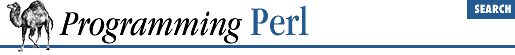home | O'Reilly's CD bookshelfs | FreeBSD | Linux | Cisco | Cisco Exam

#### 7.2.44 Math::Complex - Complex Numbers Package

```use Math::Complex;
\$cnum = new Math::Complex;```

When you use this module, complex numbers declared as:

`\$cnum = Math::Complex->new(1, 1);`

can be manipulated with overloaded math operators. The operators:

`+ - * / neg ~ abs cos sin exp sqrt`

are supported, and return references to new objects. Also,

`"" (stringify)`

is available to convert complex numbers to strings. In addition, the methods:

`Re Im arg`

are available. Given a complex number, ``` \$cnum``` :

`\$cnum = Math::Complex->new(\$x, \$y);`

then ``` \$cnum->Re()``` returns ``` \$x``` , ``` \$cnum->Im()``` returns ``` \$y``` , and ``` \$cnum->arg()``` returns ``` atan2(\$y, \$x)``` .

``` sqrt()``` , which should return two roots, returns only one.7.2.43 Math::BigInt - Arbitrary-Length Integer Math Package7.2.45 NDBM_File - Tied Access to NDBM Files